## 1. Introduction

In this tutorial, we’ll see how to compute the number of ways to arrange theblocks withvisible blocks on the left andvisible ones on the right.

## 2. Problem Description

There areuniquely-sized blocks whose heights are integers fromto. How many ways to arrange theblocks in a row such that exactlyblocks are visible from the left andblocks are visible from the right?

A block is visible from the left if there are no longer blocks to the left of it. Similarly, a block is visible from the right if there are no longer blocks to the right of it.

For example, if we arrangeblocks with, then the blocks with heights,, andare visible from the left. Also, blocks with heightsandare visible from the right: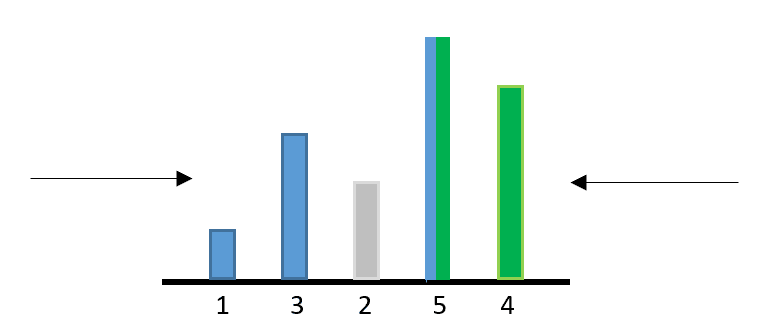The longest block with the height ofis visible from both sides.

## 3. Solve the Left Part

Let’s first consider the left part only. Givenblocks, letdenote the number of ways to arrange them withvisible blocks from the left. We can divide this problem into two cases.

For the first case, we place the longest block in the end. In this way, the longest block is always visible from the left no matter how we arrange the restblocks. Therefore, the problem becomes to solveas we need to find the number of ways to arrange the remainingblocks withvisible ones: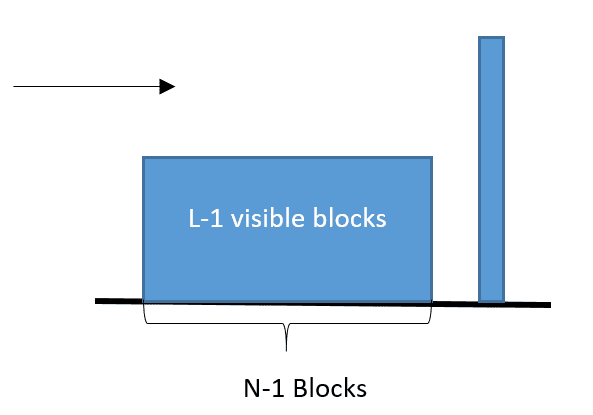For the second case, we place any block other than the longest one in the end. Since the longest block is not at the end position, it hides any blocks right after it, including the last one. Therefore, we should not count the last block as the visible block from the left. To makevisible blocks from the left, we have to arrange them on the remainingblocks. Therefore, the problem becomes to solveto arrange the remainingblocks withvisible ones: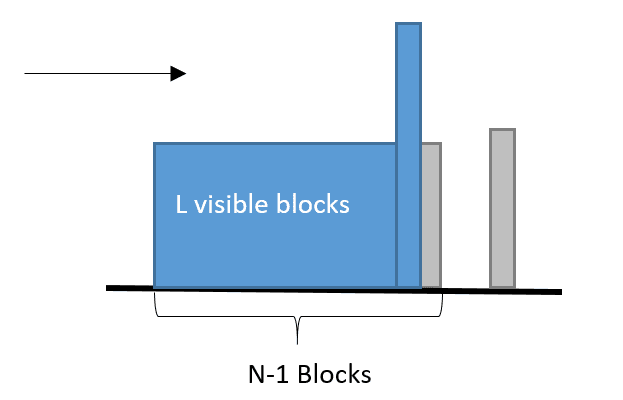Since we havepossible ways to put a short block into the last position, the total number of ways for the second case is.

Based on the above observations, we can have our recurrence formula:For the base case, if we have only one block, then there is only one way to see it visible from the left. Therefore, we can have. Also, we havefor.

We can use a dynamic programming approach to compute the:This algorithm uses a two-dimensional array,, to compute the. The array hasrows andcolumns, as the array index starts from. We use this array to store the computedvalues. When we computewith biggerand, we can reuse the previously computed results from the smaller numbers.

Firstly, we store the base case,, into thearray. Then, we use nested loops to go through all possibleandvalues and computebased on our recurrence formula. After we finish the computing,is the result we want.

Since we have two nested loops over the numbersand, the overall time complexity of this algorithm is.

## 4. S0lve Both Parts

Let’s consider an arrangement that containsvisible blocks from the left andvisible blocks from the right. For each visible block, we treat this block and all blocks between it and the next visible block as a whole segment. The longest block is a segment that contains itself only. Also, it is visible from both sides. Therefore, there aresegments before the longest block segment andsegments after the longest block segments: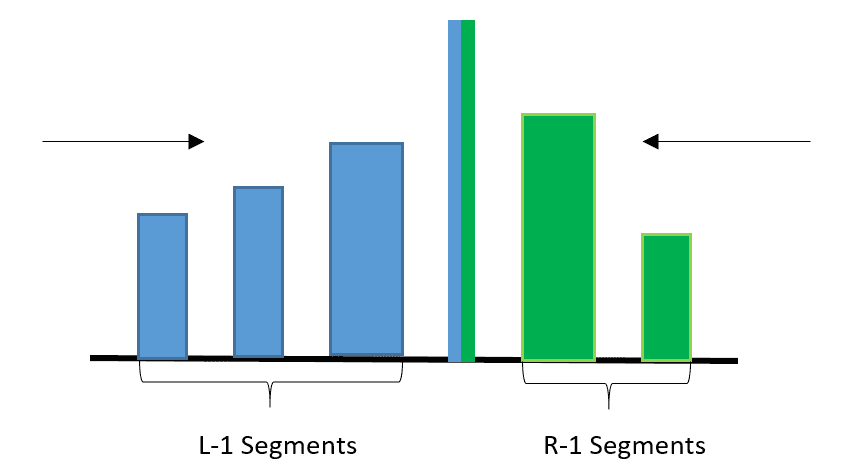For each segment on the right side, we can reverse the order of the blocks and move the whole segment to the left side. This makessegments before the longest block. Also, we can sort these segments based on the highest block in each segment. In this way, we can havevisible blocks to the left of the longest block: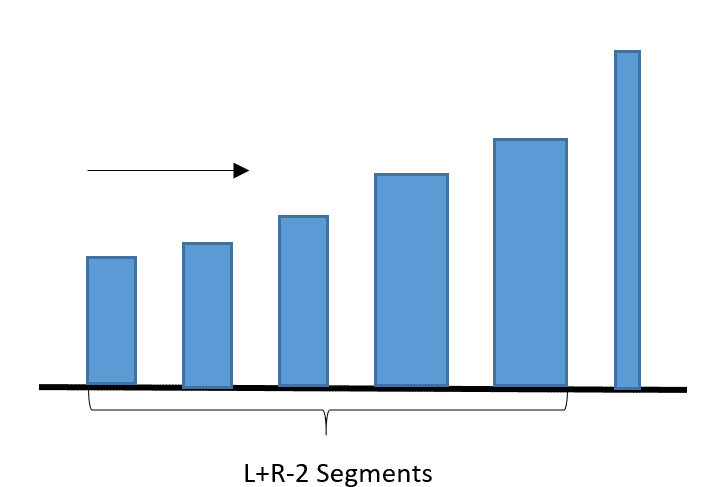Similarly, given a block arrangement where the longest block is at the last position and there arevisible blocks from the left, we can picksegments and move them to the right of the longest block. In this way, we can have a block arrangement that containsvisible blocks from the left andvisible blocks from the right.

Therefore, we can reduce the original problem to a smaller problem that computes the arrangement where the longest block is at the end position and there arevisible blocks among the restblocks, which is.

Also, among thesegments, we can choose anysegments and move them to the right. Therefore, the overall number of possible ways is, which is also.

We can use a factorial function and the previousfunction to calculate our result:The time complexity for the factorial functions is. Also, thefunction takestime. Therefore, the overall time complexity of this algorithm is## 5. Conclusion

In this tutorial, we showed a dynamic programming algorithm to compute the number of arrangements forblocks withvisible blocks from the left. Based on this algorithm, we can extend to compute the number of arrangements forblocks withvisible blocks from the left andvisible blocks from the right.